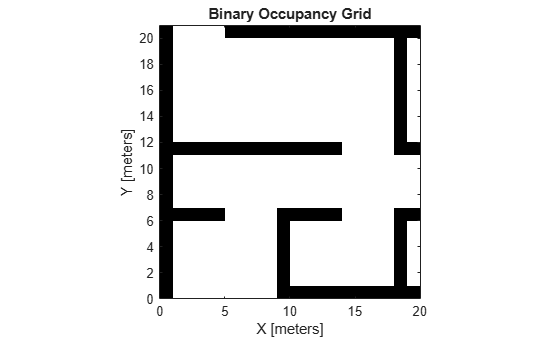# show

Show occupancy grid values

## Syntax

``show(map)``
``show(map, "local")``
``show(map, "grid")``
``show(___,Name,Value)``
``mapImage = show(___)``

## Description

````show(map)` displays the binary occupancy grid `map` in the current axes, with the axes labels representing the world coordinates.```
````show(map, "local")` displays the binary occupancy grid `map` in the current axes, with the axes labels representing the local coordinates instead of world coordinates.```
````show(map, "grid")` displays the binary occupancy grid `map` in the current axes, with the axes labels representing the grid coordinates.```

example

````show(___,Name,Value)` specifies additional options specified by one or more name-value pair arguments.```
````mapImage = show(___)` returns the handle to the image object created by `show`.```

## Examples

collapse all

This example shows how to move a local egocentric map and sync it with a larger world map. This process emulates a vehicle driving in an environment and getting updates on obstacles in the new areas.

Load example maps. Create a binary occupancy map from the `complexMap`.

```load exampleMaps.mat map = binaryOccupancyMap(complexMap); show(map)```Create a smaller local map.

```mapLocal = binaryOccupancyMap(complexMap(end-20:end,1:20)); show(mapLocal)```Follow a path planned in the world map and update the local map as you move your local frame.

Specify path locations and plot on the map.

```path = [5 2 8 2 8 8 30 8]; show(map) hold on plot(path(:,1),path(:,2)) hold off```Create a loop for moving between points by the map resolution. Divide the difference between points by the map resolution to see how many incremental moves you can make.

```for i = 1:length(path)-1 moveAmount = (path(i+1,:)-path(i,:))/map.Resolution; for j = 1:abs(moveAmount(1)+moveAmount(2)) moveValue = sign(moveAmount).*map.Resolution; move(mapLocal,moveValue, ... "MoveType","relative","SyncWith",map) show(mapLocal) drawnow limitrate pause(0.2) end end```## Input Arguments

collapse all

Map representation, specified as a `binaryOccupancyMap` object. This object represents the environment of the vehicle.

### Name-Value Arguments

Specify optional pairs of arguments as `Name1=Value1,...,NameN=ValueN`, where `Name` is the argument name and `Value` is the corresponding value. Name-value arguments must appear after other arguments, but the order of the pairs does not matter.

Before R2021a, use commas to separate each name and value, and enclose `Name` in quotes.

Example: `'Parent',axHandle`

Axes to plot the map specified as either an `Axes` or `UIAxes`object. See `axes` or `uiaxes`.

Update existing map plot, specified as `0` or `1`. If you previously plotted your map on your figure, set to `1` for a faster update to the figure. This is useful for updating the figure in a loop for fast animations.

## Version History

Introduced in R2015a